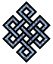#fail2ban bad ip database: ip 188.152.254.191

### | ip database | live view | stats | report | help | api key:

 ip: 188.152.254.191 hostname: 188.152.254.191 country:[IT] Italy first reported: 18.08.2018 04:09.29 GMT+0200 last reported: 11.01.2019 18:57.09 GMT+0200 time period: 146d 15h 47m 40s total reports: 6 reported by: 3 host(s) filter(s): ssh (5) ssh (1) tor exit node no badips.com db Lookup## port scan of '188.152.254.191':

[-hide]
```# Nmap 6.40 scan initiated Sat Aug 18 04:10:03 2018 as: /usr/bin/nmap -sU -sS -O 188.152.254.191
Nmap scan report for 188.152.254.191
Host is up (0.035s latency).
Not shown: 998 open|filtered ports, 991 closed ports
PORT     STATE    SERVICE
20/tcp   filtered ftp-data
21/tcp   filtered ftp
22/tcp   filtered ssh
23/tcp   filtered telnet
80/tcp   filtered http
179/tcp  filtered bgp
443/tcp  open     https
3389/tcp filtered ms-wbt-server
4000/tcp filtered remoteanything
123/udp  open     ntp
161/udp  open     snmp
No exact OS matches for host (If you know what OS is running on it, see http://nmap.org/submit/ ).
TCP/IP fingerprint:
OS:SCAN(V=6.40%E=4%D=8/18%OT=443%CT=1%CU=%PV=N%G=Y%TM=5B778145%P=x86_64-pc-
OS:linux-gnu)SEQ(SP=106%GCD=1%ISR=10B%TI=I%CI=I%TS=U)SEQ(SP=107%GCD=1%ISR=1
OS:09%TI=I%TS=U)OPS(O1=M5B4%O2=M5B4%O3=M5B4%O4=M5B4%O5=M5B4%O6=M5B4)WIN(W1=
OS:FFFF%W2=FFFF%W3=FFFF%W4=FFFF%W5=FFFF%W6=FFFF)ECN(R=Y%DF=N%TG=FF%W=FFFF%O
OS:=M5B4%CC=N%Q=)T1(R=Y%DF=N%TG=FF%S=O%A=S+%F=AS%RD=0%Q=)T2(R=N)T3(R=N)T4(R
OS:=Y%DF=N%TG=FF%W=FFFF%S=A%A=Z%F=R%O=%RD=0%Q=)T5(R=Y%DF=N%TG=FF%W=0%S=Z%A=
OS:S+%F=AR%O=%RD=0%Q=)T6(R=Y%DF=N%TG=FF%W=0%S=A%A=Z%F=R%O=%RD=0%Q=)T7(R=Y%D
OS:F=N%TG=FF%W=0%S=Z%A=S%F=AR%O=%RD=0%Q=)U1(R=N)IE(R=Y%DFI=S%TG=FF%CD=S)

OS detection performed. Please report any incorrect results at http://nmap.org/submit/ .
# Nmap done at Sat Aug 18 04:15:33 2018 -- 1 IP address (1 host up) scanned in 331.81 seconds
```
```Σ = 61 | Δt = 0.0047290325164795s
```